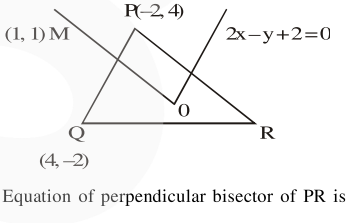# In a triangle PQR,

Question:

In a triangle PQR, the co-ordinates of the points $P$ and $Q$ are $(-2,4)$ and $(4,-2)$ respectively. If the equation of the perpendicular bisector of PR is $2 x-y+2=0$, then the centre of the circumcircle of the $\triangle \mathrm{PQR}$ is :

1. $(-1,0)$

2. $(-2,-2)$

3. $(0,2)$

4. $(1,4)$

Correct Option: , 2

Solution:Solving with $2 x-y+2=0$ will give $(-2,2)$